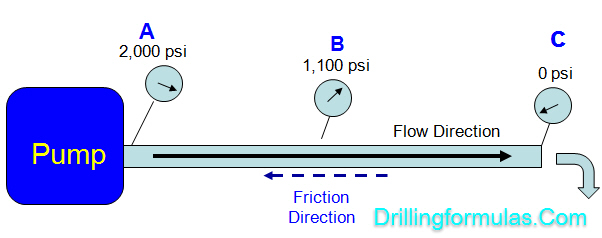## Understand Pressure Loss (Frictional Pressure) in Drilling System

Frictional pressure is pressure loss acting in the opposite direction of fluid flow and today we will look into each component in pressure therefore you will get clearer picture about the frictional pressure in drilling system.

Let take a look at the simple diagram below.A mud pump creates power to move drilling fluid from point A to C and the frictional pressure or pressure loss is the amount of pressure required to transfer fluid.

Pump pressure is 2,000 psi at the starting point (“A”) and at the end point (“C”), pressure is 0 psi. This tells you that you need 2,000 psi to overcome the frictional pressure in order to move the fluid from point “A” to point “C”.

“Differential pressure between two points in the system is pressure loss while fluid is moved from one to another point.”

## Pressure Loss and Equivalent Circulating Density Review

This example that I got from my junior member is very simple but it helps you a lot to understand about how to determine pressure loss during normal circulation.

Information given is listed below;Circulate at 3 bottom up through open end tubing (Down tubing and up annulus) with 12.7 ppg mud.

Pump pressure = 1000 psi

Annulus friction loss = 50 psi

Inside tubing friction loss = 925 psi

Surface line friction loss = 25 psi